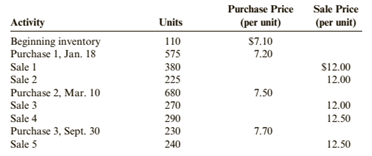Chapter 6, Problem 50E### Cornerstones of Financial Accounti...

4th Edition
Jay Rich + 1 other
ISBN: 9781337690881

#### Solutions

Chapter
Section### Cornerstones of Financial Accounti...

4th Edition
Jay Rich + 1 other
ISBN: 9781337690881
Textbook Problem
10 views

# Inventory Costing MethodsCrandall Distributors uses a perpetual inventory system and has the following data available for inventory, purchases, and sales for a recent year.Required:1. Compute the cost of ending inventory and the cost of goods sold using the specific identification method. Assume the ending inventory is made up of 40 units from beginning inventory, 30 units from Purchase 1, 80 units from Purchase 2, and 40 units from Purchase 3.2. Compute the cost of ending inventory and cost of goods sold using the FIFO inventory costing method.3. Compute the cost of ending inventory and cost of goods sold using the LIFO inventory costing method.4. Compute the cost of ending inventory and cost of goods sold using the average cost inventory costing method. ( Note: Use four decimal places for per-unit calculations and round all other numbers to the nearest dollar.)5. CONCEPTUAL CONNECTION Compare the ending inventory and cost of goods sold computed under all four methods. What can you conclude about the effects of the inventory costing methods on the balance sheet and the income statement?

To determine

(a)

Inventory costing methods:

FIFO, LIFO and average cost method, are those method which used for calculation of closing inventory and cost of goods sold.

The cost of ending inventory and the cost of goods sold using the specific identification method.

Explanation

The given information is as follows:

Total available units are:

Opening inventory=110 units @ $7.10 each Purchases=575 units @$7.20 eachPurchases=680 units @ $7.50 eachPurchases=230 units @$7.70 each

Total Purchased units = 1485

Total available quantity = (1485+110)= 1595 units

Sales=1405 units

Closing inventory =Beginning inventory + PurchaseSales=110+14851405=190 units

Calculation of Closing Inventory as per specific identification Method:

Under this method, closing inventory is provided to be valued.

 Closing inventory Cost of Goods sold 70 units @ \$7
To determine

(b)

Inventory costing methods:

FIFO, LIFO and average cost method, are those method which used for calculation of closing inventory and cost of goods sold.

The cost of ending inventory and the cost of goods sold using the FIFO.

To determine

(c)

Inventory costing methods:

FIFO, LIFO and average cost method, are those method which used for calculation of closing inventory and cost of goods sold.

The cost of ending inventory and the cost of goods sold using the LIFO.

To determine

(d)

Inventory costing methods:

FIFO, LIFO and average cost method, are those method which used for calculation of closing inventory and cost of goods sold.

The cost of ending inventory and the cost of goods sold using the average cost method.

To determine

(e)

Inventory costing methods:

FIFO, LIFO and average cost method, are those method which used for calculation of closing inventory and cost of goods sold.

The comparison of ending inventory and cost of goods sold and comment the effects of these on income statement and balance sheet.

### Still sussing out bartleby?

Check out a sample textbook solution.

See a sample solution

#### The Solution to Your Study Problems

Bartleby provides explanations to thousands of textbook problems written by our experts, many with advanced degrees!

Get Started

#### Find more solutions based on key concepts